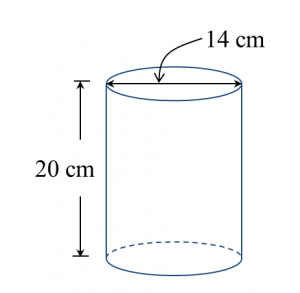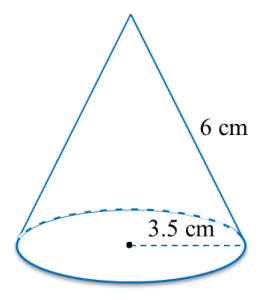# 6.4.1 Surface Area of Three Dimensional Shapes, PT3 Focus Practice

6.4.1 Surface Area of Three Dimensional Shapes, PT3 Focus Practice

Question 1:
Diagram below shows closed right cylinder.Calculate the total surface area, in cm2, of the cylinder.

$\left(\pi =\frac{22}{7}\right)$

Solution
:
Total surface area
= 2(πr2) + 2πrh
$\begin{array}{l}=\left(2×\frac{22}{7}×{7}^{2}\right)+\left(2×\frac{22}{7}×7×20\right)\\ =308+880\\ =1188c{m}^{2}\end{array}$

Question 2:
Diagram below shows a right prism with right-angled triangle ABC as its uniform cross section.Calculate the total surface area, in cm2, of the prism.

Solution
:
$\begin{array}{l}AB=\sqrt{{5}^{2}-{3}^{2}}\\ =\sqrt{25-9}\\ =\sqrt{16}\\ =4cm\end{array}$

Total surface area
= 2 (½× 3 × 4) + (3 × 10) + (4 × 10) + (5 × 10)
= 12 + 30 + 40 + 50
= 132 cm2

Question 3:The diagram shows a cone. The radius of its base is 3.5 cm and its slant height is 6 cm. Find the area of its curved surface.
( π= 22 7 )

Solution
:
Area of curved surface
= π × radius of base × slant height
= πrs
$\begin{array}{l}=\frac{22}{7}×3.5×6\\ =66c{m}^{2}\end{array}$